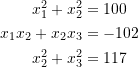### Mathematica Lab Tutorials: Lab 6

### Lab Questions

Create a procedure whose inputs are the vector of functions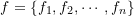, the vector of the names of the unknowns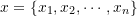and the initial guesses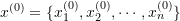. The procedure should do the following:

• Find the length of the vectors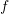or(These should be equal, a more robust code should stop the computations if they are not but this is not required)
• The procedure should then form the matrix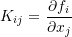• The procedure should then output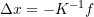evaluated at the values of the initial guesses.

Use the above procedure along with an appropriate while loop to find two different solutions (using two different initial guesses) for the following system of nonlinear equations. Use ε_s=0.00001. Compare with the Newton Raphson method implemented in Mathematica using (FindRoot).